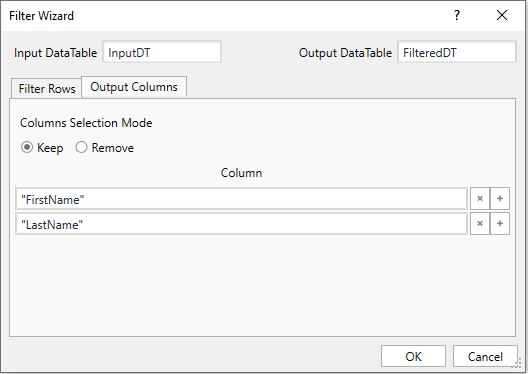### Filter DataTable in UiPath StudioUiPath - Filter DataTable

It is very important to be able to perform filter operations on DataTable in order to get a desired result.

Let’s take an example of having a table with columns such as FirstName, LastName and Age. We need to filter the records having FirstName as "David".

Input DataTable variable – InputDT
Output Filtered DataTable variable – FilteredDT

Method 1 – Using "Filter Data Table" Activity

Add the variables and conditions as shown below:Filter DataTable Activity

We can also choose the columns needed as a resulted FilteredDT, by adding columns via the Output Columns Tab as shown below:Filter DataTable Activity - Keep/Remove Output Columns

Method 2 – Using "DataTable.Select() method"

Using this method, we can directly assign filtered rows to a DataRow array or DataTable using below given expression. By default, this method returns the array of data rows, but we can convert it to DataTable.

FilteredDT = InputDT.Select("[FirstName]='John'").CopyToDataTable

Method 3 – Using "DataTable.AsEnumerable() method"

Just write the expression as given below:

FilteredDT = InputDT.AsEnumerable().Where(function(f) f(“FirstName”).ToString=”David”).CopyToDataTable

Method 4 – Using "LINQ"

LINQ method returns IEnumerable by default.

FilteredDT = (From r In InputDT.Select()
Where r("FirstName").Equals("David")
Select r).CopyToDataTable